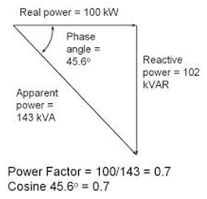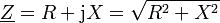Deutsche Version● The Reactance X is the imaginary Part
of the complex
Impedance
Z

Calculation of Capacity C and Inductivity L

Enter any two known values and press "Calculate" to solve for the others.
For example, a 1 μF capacitor or a 25.3 mH inductor will have 159 ohms of reactance
at a frequency of 1000 Hertz. Press "reset" before doing a new calculation.

 capacitance C  in microfarads inductance L  in millihenrys reactance X  in ohms frequency f0  in hertz

Inductive reactance XL = 2 · π · f · L
Capacitive reactance XC = 1 / (2 · π · f · C)
Resonant frequency f0 = 1 / (2 · π ·√(L · C))

Capacitive reactance calculator XC

 A typical question: "How to measure the inductance of a coil?" http://lmgtfy.com/?q=How+to+measure+inductance?   A typical question: "How to measure the capacitance of a capacitor?" http://lmgtfy.com/?q=How+to+measure+capacitance?Apparent resistance (impedance):Impedance Z = √ (electrical reactance X)² + (effective resistance R)². Apparent power S = √ (reactive Q2 + real power P2).

 Real power P in watts Reactive Power Q in volt-amps reactive (VAR) Apparent power (complex power) S in (VA) volt-amps S = √ (Q² + P²) = √ (reactive power²+ real power²)